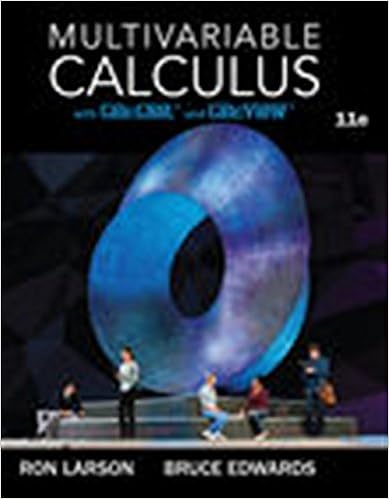# Perpendicular lines form right angles 5 5 6 6 7 7

• Homework Help
• 36

This preview shows page 16 - 24 out of 36 pages.

##### We have textbook solutions for you!
The document you are viewing contains questions related to this textbook.The document you are viewing contains questions related to this textbook.
Chapter 13 / Exercise 110
Multivariable Calculus
LarsonExpert Verified
1) ? 1) ? 2) ??≅ ??2) ? 3) ??≅ ??3) ? 4) ? 4) Perpendicular lines form right angles 5) ∠??? ≅ ∠???5) ? 6) ∆??? ≅ ∆???6) ? 7) ? 7) ? Statements/Reasons Bank: CPCTC Vertical angles are congruent ??≅ ??All right angles are congruent Given Reflexive property of SSS Triangle Congruence ??≅ ??SAS Triangle Congruence ∠? ≅ ∠?ASA Triangle Congruence ∠??? ≅ ∠???AAS Triangle Congruence ∠??? & ∠???are right angles HL Triangle Congruence Transitive property of
##### We have textbook solutions for you!
The document you are viewing contains questions related to this textbook.The document you are viewing contains questions related to this textbook.
Chapter 13 / Exercise 110
Multivariable Calculus
LarsonExpert Verified
Geometry-Congruent Triangles ~17~NJCTL.orgCPCTC Honors Classwork For numbers 73 74 state the reason the two triangles are congruent. Then list all other corresponding parts of the triangles that are congruent.73. 74. Given:HIJGPARCC-type problems 75.Complete the proof. Given:GKistheperpendicularbisectorofFH.Prove: FGHGStatementsReasons1) GKis the perpendicular bisector of FH.1) 2)2)Def. of perpendicular bisector3) GKF GKH3)All right are .4)4)Reflexive Prop. of 5)∆FGK ∆HGK5)6)6)CPCTC
Geometry-Congruent Triangles ~18~NJCTL.org76. Write a proof. Given: YABA, B Y Prove: AZACStatementsReasons
Geometry-Congruent Triangles ~19~NJCTL.orgCPCTC Honors Homework For numbers 77 78 state the reason the two triangles are congruent. Then list all other corresponding parts of the triangles that are congruent.77. ZXWand ∆YWX78. ∆ABE and ∆ACDPARCC-type problems 79. Complete the proof.Given:ABCEisarectangle; D is the midpoint ofCE. Prove: ADBDStatementsReasons1)ABCE is a rectangle. D is the midpoint of CE.1)Given2)AED BCD2)Definition of rectangle3)AEBC3)Definition of rectangle4)4)5)5)6)6)
Geometry-Congruent Triangles ~20~NJCTL.org80. Write a proof. Given: BDAC, D is the midpoint of AC.Prove: BCBAStatementsReasons
Geometry-Congruent Triangles ~21~NJCTL.orgIsosceles and Equilateral Triangles Classwork Complete the statement using ALWAYS, SOMETIMES, and NEVER. 81. An isosceles triangle is ___________ a scalene triangle. 82. An equilateral triangle is __________ an isosceles triangle. 83. An isosceles triangle is ___________ an equilateral triangle. 84. An acute triangle is ___________ an equiangular triangle. 85. An isosceles triangle is __________ a right triangle. Solve for each variable in exercises 86 94. Figures are not drawn to scale. 86.87.88.89.90.91.92.93.94.
Geometry-Congruent Triangles ~22~NJCTL.orgIsosceles and Equilateral Triangles Homework Complete the statement using ALWAYS, SOMETIMES, and NEVER. 95. A scalene triangle is ___________ an equilateral triangle. 96. An equilateral triangle is __________ an obtuse triangle. 97. An isosceles triangle is ___________ an acute triangle. 98. An equiangular triangle is ___________ a right triangle. 99. A right triangle is __________ an isosceles triangle. Solve for each variable in exercises 100 108. Figures are not drawn to scale100.101.102.103.104.105.106.107.108.
Geometry-Congruent Triangles ~23~NJCTL.orgCongruent Triangles - Unit Review PMI Geometry Multiple Choice Circle the correct answer 1. In the given triangle, find x and y.
•••